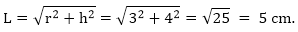Click to Chat

1800-1023-196

+91-120-4616500

CART 0

• 0

MY CART (5)

Use Coupon: CART20 and get 20% off on all online Study Material

ITEM
DETAILS
MRP
DISCOUNT
FINAL PRICE
Total Price: Rs.

There are no items in this cart.
Continue Shopping• Complete JEE Main/Advanced Course and Test Series
• OFFERED PRICE: Rs. 15,900
• View Details

```Chapter 16: Understanding Three Dimensional Shapes Exercise 16.2

Question: 1

Give two new examples of each of the for three-dimensional shapes:

(i) Cone

(ii) Sphere

(iii) Cylinder

(iv) Cuboid

(v)  Pyramid

Solution:

(i) A school bell and a funnel.

(ii) A tennis ball and a model of a globe.

(iii) Drink cans and delivering pipes for a water and gas.

(iv) A match box and brick.

(v) A paper- weight and a tower like the Eiffel tower.

Question: 2

What is the shape of:

(i) instrument box

(ii) a brick

(iii) a match box

(iv) a rod- roller

Solution:

(i) My instrument box is in the shape of a cuboid.

(ii) A brick is in the shape of the cuboid.

(iii) A match – box is in the shape of a cuboid.

(iv) A road – roller is in the shape of a cylinder.

(v) A sweet laddoo is shaped like a sphere.

Objective Type Questions:

Question: 1

Total number of faces of a cuboid is

(a) 4

(b) 6

(c) 8

(d) 12

Solution:

(b) 6

Question: 2

Total number of edges of a cuboid is

(a) 4

(b) 6

(c) 8

(d) 12

Solution:

(d) 6

Question: 3

Number of faces of a cuboid is

(a) 4

(b) 6

(c) 8

(d) 12

Solution:

(c) 8

Question: 4

Which one of them is example of cuboid?

(a) a dice

(b) a football

(c) a gas pipe

(d) an ice- cream cone

Solution:

(a) A dice

Question: 5

A brick is an example of

(a) cube

(b) cuboid

(c) prism

(d) cylinder

Solution:

(b) Cuboid

Question: 6

A gas pipe is an example of

(a) cone

(b) a cylinder

(c) cube

(d) sphere

Solution:

(b) A cylinder

Question: 7

If the base radius and height of a right circular cone are 3 cm and 4 cm in lengths, then the slant height is

(a) 5 cm

(b) 2 cm

(c) 25 cm

(d) 6 cm

Solution:

(a) 5 cmQuestion: 8

The number of faces of a triangular pyramid is

(a) 3

(b) 4

(c) 6

(d) 8

Solution:

(b) 4

A pyramid is called a triangular pyramid if its base is a triangle.

Question: 9

The number of edges of a triangular pyramid is

(a) 3

(b) 4

(c) 6

(d) 8

Solution:

(c) 6

Question: 10

A tetrahedron is a pyramid whose base is a

(a) triangle

(b) square

(c) rectangle

Solution:

(a) Triangle
```### Course Features

• 728 Video Lectures
• Revision Notes
• Previous Year Papers
• Mind Map
• Study Planner
• NCERT Solutions
• Discussion Forum
• Test paper with Video Solution